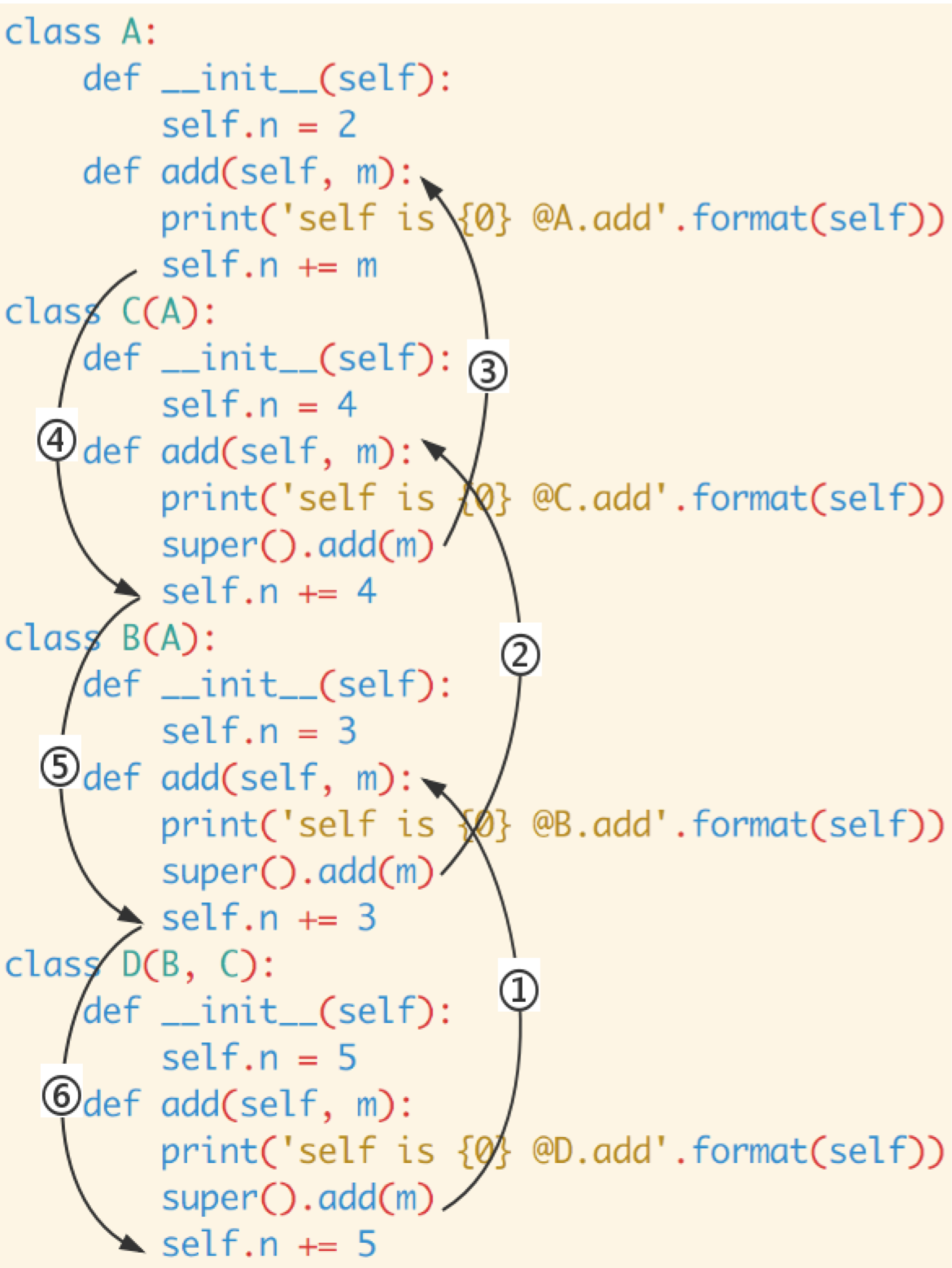# Python: super 没那么简单

## 约定¶

```# 默认， Python 3
class A:
pass

# Python 2
class A(object):
pass
```

Python 3 和 Python 2 的另一个区别是: Python 3 可以使用直接使用 super().xxx 代替 super(Class, self).xxx :

```# 默认，Python 3
class B(A):

# Python 2
class B(A):
```

## 单继承¶

```class A:
def __init__(self):
self.n = 2

self.n += m

class B(A):
def __init__(self):
self.n = 3

self.n += 3
```

```b = B()
print(b.n)
```

```self is <__main__.B object at 0x106c49b38> @B.add
self is <__main__.B object at 0x106c49b38> @A.add
8
```

2. super().add(m) 调用父类方法 def add(self, m) 时, 此时父类中 self 并不是父类的实例而是子类的实例, 所以 super().add(m) 之后 self.n 的结果是 5 而不是 4

## 多继承¶

```class C(A):
def __init__(self):
self.n = 4

self.n += 4

class D(B, C):
def __init__(self):
self.n = 5

self.n += 5
```

```d = D()
print(d.n)
```

```self is <__main__.D object at 0x10ce10e48> @D.add
self is <__main__.D object at 0x10ce10e48> @B.add
self is <__main__.D object at 0x10ce10e48> @C.add
self is <__main__.D object at 0x10ce10e48> @A.add
19
```

```self is <__main__.D object at 0x10ce10e48> @D.add
self is <__main__.D object at 0x10ce10e48> @B.add
self is <__main__.D object at 0x10ce10e48> @A.add
15
```

## super 是个类¶

```>>> class A: pass
...
>>> s = super(A)
>>> type(s)
<class 'super'>
>>>
```

```super(a_type, obj)
```

MRO 列表指的是 type(obj) 的 MRO 列表, MRO 中的那个类就是 a_type , 同时 isinstance(obj, a_type) == True

```super(type1, type2)
```

MRO 指的是 type2 的 MRO 列表, MRO 中的那个类就是 type1 ，同时 issubclass(type2, type1) == True

```[A, B, C, D, E, object]
```

```super(C, A).foo()
```

super 只会从 C 之后查找，即: 只会在 DEobject 中查找 foo 方法。

## 多继承中 super 的工作方式¶

```d = D()
print(d.n)
```

```self is <__main__.D object at 0x10ce10e48> @D.add
self is <__main__.D object at 0x10ce10e48> @B.add
self is <__main__.D object at 0x10ce10e48> @C.add
self is <__main__.D object at 0x10ce10e48> @A.add
19
```

• D 的 MRO 是: [D, B, C, A, object]备注: 可以通过 D.mro() (Python 2 使用 D.__mro__ ) 来查看 D 的 MRO 信息）, MRO 的计算方法可以参考之前写的 一篇文章
• 详细的代码分析如下:
```class A:
def __init__(self):
self.n = 2

# 第四步
# self == d, self.n == d.n == 5
self.n += m
# d.n == 7

class B(A):
def __init__(self):
self.n = 3

# 第二步
# self == d, self.n == d.n == 5
# self 的 MRO 是 [D, B, C, A, object]
# 从 B 之后的 [C, A, object] 中查找 add 方法

# 第六步
# d.n = 11
self.n += 3
# d.n = 14

class C(A):
def __init__(self):
self.n = 4

# 第三步
# self == d, self.n == d.n == 5
# self 的 MRO 是 [D, B, C, A, object]
# 从 C 之后的 [A, object] 中查找 add 方法

# 第五步
# d.n = 7
self.n += 4
# d.n = 11

class D(B, C):
def __init__(self):
self.n = 5

# 第一步
# self 的 MRO 是 [D, B, C, A, object]
# 从 D 之后的 [B, C, A, object] 中查找 add 方法

# 第七步
# d.n = 14
self.n += 5
# self.n = 19

d = D()
print(d.n)
```

```D.mro() == [D, B, C, A, object]
d = D()
d.n == 5

class D(B, C):          class B(A):            class C(A):             class A:
self.n += 5   <------6. self.n += 3    <----5. self.n += 4     <----4. <--|
(14+5=19)               (11+3=14)              (7+4=11)                (5+2=7)
```## 实现一个 Super 类¶

super() 实际上做了啥呢？简单来说就是：提供一个 MRO 列表以及一个 MRO 中的类 C ， super() 将返回一个从 MRO 列表中 C 之后的类中查找方法的对象。

```from functools import partial

class Super:
def __init__(self, sub_cls, instance):
# 假设 sub_cls = B, instance = D()
mro = instance.__class__.mro()
# mro == [D, B, C, A, object]
# sub_cls is B
# 从 mro 中 sub_cls 后面的类中进行查找
# __mro_tail == [C, A, object]
self.__mro_tail = mro[mro.index(sub_cls)+1:]
self.__sub_cls = sub_cls
self.__instance = instance

def __getattr__(self, name):
# 从 mro tail 列表的各个类中查找方法
for cls in self.__mro_tail:
if not hasattr(cls, name):
continue

print('call {}.{}'.format(cls, name))
# 获取类中定义的方法
attr = getattr(cls, name)
# 所以返回的函数需要自动填充第一个 self 参数
return partial(attr, self.__instance)

raise AttributeError(name)
```

```class A:
def __init__(self):
self.n = 2

self.n += m

class B(A):
def __init__(self):
self.n = 3

self.n += 3

class C(A):
def __init__(self):
self.n = 4

self.n += 4

class D(B, C):
def __init__(self):
self.n = 5

self.n += 5

d = D()
print(d.n)
```

```self is <__main__.D object at 0x10d02cf98> @D.add
self is <__main__.D object at 0x10d02cf98> @B.add
self is <__main__.D object at 0x10d02cf98> @C.add
self is <__main__.D object at 0x10d02cf98> @A.add
19
```